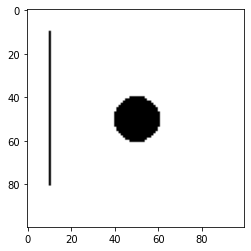# Pytorch binary tensor

Thank you very much for reading my question . A newbie here sorry if my question isnt asked quite right

for example I have this image and want to convert it to binary tensor that only has 1s and 0s, I was just using

``````import torch
import numpy as np
from PIL import Image
from torchvision import transforms
import torchvision.transforms.functional as fn

cc = Image.open("C:/Users/T/Desktop/circle.png")
cc = fn.resize(cc, size=[300,200])

tt = transforms.ToTensor()
gg = transforms.Grayscale()
circle_tensor = gg(tt(cc))
circle_np = circle_tensor.numpy()
pd.DataFrame(circle_np).to_csv("C:/Users/T/Desktop/circle.csv")
``````

and it becomes a tensor that has only 1 representing white and 0 representing black.

here is another example of a line ,

`I was just wondering , instead of converting an image into a tensor ,`

`if I knew the geometric coordinates of the shape in the image . ie , the center of the circle and its radius , and for the line , the two ends points [x,y], [x1,y1] coordinates . can I create this binary tensor directly within pytorch. `

ie through something like a for loop or more efficient method

``````zz = torch.zeros(300,200)

for x in range(zz.shape):
...

and some for loop ? at the moment Im using drawing it as image first and convert in this way which doesnt feel quite right in my specific situation where I know the coordinates,
``````

Thank you very much for your time in reading and helping out.!

You could certainly reimplement these drawing functions, but if you don’t need to differentiate through them I would recommend to use already available and tested libraries such as OpenCV.

Here is a small example of drawing a circle and a line into a numpy array using OpenCV and then transforming this image to a tensor:

``````import cv2
import torch
import matplotlib.pyplot as plt

# circle
# empty image
img = np.ones((100, 100))
# draw circle
c = cv2.circle(img=img, center=[50, 50], radius=10, color=0, thickness=-1, lineType=cv2.FILLED)

# line
c = cv2.line(img=c, pt1=[10, 10], pt2=[10, 80], color=0)

# visualize
plt.imshow(c, cmap="gray")

# transform to tensor
tensor = torch.from_numpy(c)
``````

Output:1 Like

hi, thank you very much for your time and effort in replying my question ! may I please also just shout out a thank you to the community and the pros with enough kindness to be willing to spend time n help out. you may have literally just saved few hours or even days of time of a newbie who doesnt even know what keyword to search on google. Thank you very much ! ps seen ur other answers in other posts b4, truly appreciate your kindness !!!

1 Like